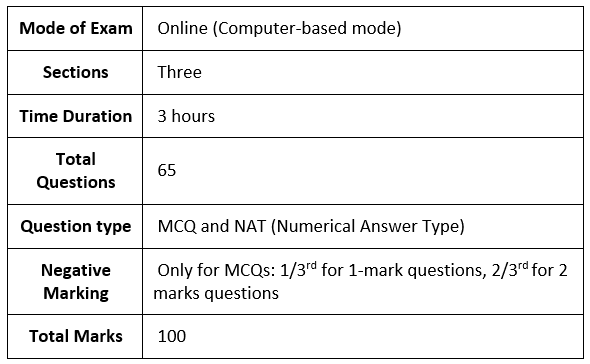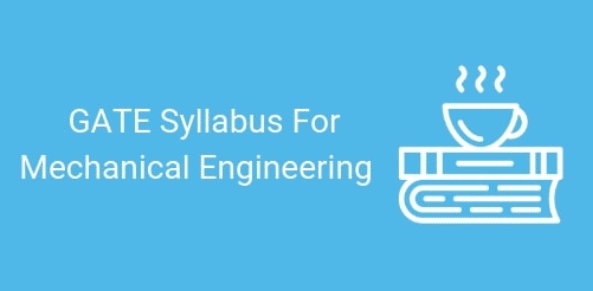# Syllabus - Mechanical Engineering: ME, GATE (Practice) Notes - GATE

## GATE: Syllabus - Mechanical Engineering: ME, GATE (Practice) Notes - GATE

The document Syllabus - Mechanical Engineering: ME, GATE (Practice) Notes - GATE is a part of GATE category.
All you need of GATE at this link: GATE

Before getting into the details of the GATE 2020 Mechanical Engineering syllabus,
Let’s have an overview of the exam:GATE 2020 for Mechanical Engineering consists of 3 sections:
(i) General Aptitude
(ii) Engineering Mathematics
(iii) Subject-specific sectionSyllabus for General Aptitude (GA) (Common to all Papers)

➢ Verbal Ability

• English grammar, sentence completion, verbal analogies, word groups, instructions, critical reasoning, and verbal deduction.

➢ Numerical Ability

• Numerical computation, numerical estimation, numerical reasoning, and data interpretation.

Mechanical Engineering

Section 1: Engineering Mathematics

1.1 Linear Algebra

• Matrix algebra, systems of linear equations, eigenvalues, and eigenvectors.

1.2 Calculus

• Functions of a single variable, limit, continuity and differentiability, mean value theorems, indeterminate forms; evaluation of definite and improper integrals; double and triple integrals; partial 64 derivatives, total derivative, Taylor series (in one and two variables), maxima, and minima, Fourier series; gradient, divergence and curl, vector identities, directional derivatives, line, surface and volume integrals, applications of Gauss, Stokes and Green’s theorems.

1.3 Differential equations

• First-order equations (linear and nonlinear); higher-order linear differential equations with constant coefficients; Euler-Cauchy equation; initial and boundary value problems; Laplace transforms; solutions of heat, wave, and Laplace's equations.

1.4 Complex variables

• Analytic functions; Cauchy-Riemann equations; Cauchy’s integral theorem and integral formula; Taylor and Laurent series.

1.5 Probability and Statistics

• Definitions of probability, sampling theorems, conditional probability; mean, median, mode and standard deviation; random variables, binomial, Poisson, and normal distributions.

1.6 Numerical Methods

• Numerical solutions of linear and non-linear algebraic equations; integration by trapezoidal and Simpson’s rules; single and multi-step methods for differential equations.

Section 2: Applied Mechanics and Design

2.1 Engineering Mechanics

• Free-body diagrams and equilibrium; trusses and frames; virtual work; kinematics and dynamics of particles and of rigid bodies in plane motion; impulse and momentum (linear and angular) and energy formulations, collisions.

2.2 Mechanics of Materials

• Stress and strain, elastic constants, Poisson's ratio; Mohr’s circle for plane stress and plane strain; thin cylinders; shear force and bending moment diagrams; bending and shear stresses; deflection of beams; torsion of circular shafts; Euler’s theory of columns; energy methods; thermal stresses; strain gauges and rosettes; testing of materials with the universal testing machine; testing of hardness and impact strength.

2.3 Theory of Machines

• Displacement, velocity, and acceleration analysis of plane mechanisms; dynamic analysis of linkages; cams; gears and gear trains; flywheels and governors; balancing of reciprocating and rotating masses; gyroscope.

2.4 Vibrations

• Free and forced vibration of single degree of freedom systems, the effect of damping; vibration isolation; resonance; critical speeds of shafts.

2.5 Machine Design

• Design for static and dynamic loading; failure theories; fatigue strength and the S-N diagram; principles of the design of machine elements such as bolted, riveted, and welded joints; shafts, gears, rolling and sliding contact bearings, brakes and clutches, springs.

Section 3: Fluid Mechanics and Thermal Sciences

3.1 Fluid Mechanics

• Fluid properties; fluid statics, manometry, buoyancy, forces on submerged bodies, the stability of floating bodies; control-volume analysis of mass, momentum, and energy; fluid acceleration; differential equations of continuity and momentum; Bernoulli’s equation; dimensional analysis; the viscous flow of incompressible fluids, boundary layer, elementary turbulent flow, flow through pipes, head losses in pipes, bends, and fittings.

3.2 Heat-Transfer

• Modes of heat transfer; one-dimensional heat conduction, resistance concept and electrical analogy, heat transfer through fins; unsteady heat conduction, lumped parameter system, Heisler's charts; thermal boundary layer, dimensionless parameters in free and forced convective heat transfer, heat transfer correlations for flow over flat plates and through pipes, the effect of turbulence; heat exchanger performance, LMTD and NTU methods; radiative heat transfer, Stefan- Boltzmann law, Wien's displacement law, black and grey surfaces, view factors, radiation network analysis.

3.3 Thermodynamics

• Thermodynamic systems and processes; properties of pure substances, the behavior of ideal and real gases; zeroth and first laws of thermodynamics, calculation of work and heat in various processes; second law of thermodynamics; thermodynamic property charts and tables, availability and irreversibility; thermodynamic relations.

3.4 Applications

• Power Engineering: Air and gas compressors; vapour and gas power cycles, concepts of regeneration and reheat. I.C. Engines: Air-standard Otto, Diesel, and dual cycles. Refrigeration and air conditioning: Vapour and gas refrigeration and heat pump cycles; properties of moist air, psychrometric 65 chart, basic psychrometric processes. Turbomachinery: Impulse and reaction principles, velocity diagrams, Pelton-wheel, Francis, and Kaplan turbines.

Section 4: Materials, Manufacturing and Industrial Engineering

4.1 Engineering Materials

• Structure and properties of engineering materials, phase diagrams, heat treatment, stress-strain diagrams for engineering materials.

4.2 Casting, Forming, and Joining Processes

• Different types of castings, design of patterns, moulds, and cores; solidification and cooling; riser and gating design. Plastic deformation and yield criteria; fundamentals of hot and cold working processes; load estimation for bulk (forging, rolling, extrusion, drawing) and sheet (shearing, deep drawing, bending) metal forming processes; principles of powder metallurgy. Principles of welding, brazing, soldering, and adhesive bonding.

4.3 Machining and Machine Tool Operations

• Mechanics of machining; basic machine tools; single and multipoint cutting tools, tool geometry and materials, tool life and wear; economics of machining; principles of non-traditional machining processes; principles of work holding, design of jigs and fixtures.

4.4 Metrology and Inspection

• Limits, fits, and tolerances; linear and angular measurements; comparators; gauge design; interferometry; form and finish measurement; alignment and testing methods; tolerance analysis in manufacturing and assembly.

4.5 Computer Integrated Manufacturing

• Basic concepts of CAD/CAM and their integration tools.

4.6 Production Planning and Control

• Forecasting models, aggregate production planning, scheduling, materials requirement planning.

4.7 Inventory Control

• Deterministic models; safety stock inventory control systems.

4.8 Operations Research

• Linear programming, simplex method, transportation, assignment, network flow models, simple queuing models, PERT, and CPM.
The document Syllabus - Mechanical Engineering: ME, GATE (Practice) Notes - GATE is a part of GATE category.
All you need of GATE at this link: GATEUse Code STAYHOME200 and get INR 200 additional OFF

Track your progress, build streaks, highlight & save important lessons and more!

,

,

,

,

,

,

,

,

,

,

,

,

,

,

,

,

,

,

,

,

,

,

,

,

;